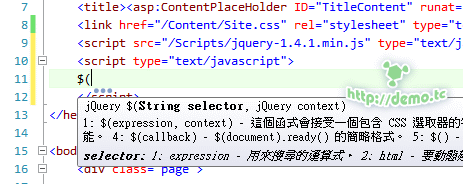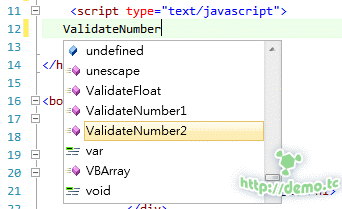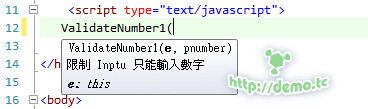# demoshop

••••••••demo, trying to be the best_

## 如何讓自己寫的 JS 也擁有 tooltip (註解)

• 2010-07-11
• 8792
• 0這就是官方提供的 jQuery智能提示（Intellisense）還中文的呢。假設我們自己寫了一個 JS 通常都會利用註解的方式來說明這 Function 是做什麼的。

```//Input 只能輸入數字
function ValidateNumber1(e, pnumber)
{
if (!/^\d+\$/.test(pnumber))
{
e.value = /^\d+/.exec(e.value);
}
return false;
}

//Input 只能輸入數字
function ValidateNumber2(e, pnumber)
{
if (!/^\d+[.]?\d*\$/.test(pnumber))
{
e.value = /^\d+[.]?\d*/.exec(e.value);
}
return false;
}

//Input 只能輸入數字可以有小數點
function ValidateFloat(e, pnumber)
{
if (!/^\d+[.]?[1-9]?\$/.test(pnumber))
{
e.value = /\d+[.]?[1-9]?/.exec(e.value);
}
return false;
}```在實際使用上，JS檔的註解根本沒意義.....所以要利用 Visual Studio 看得懂的註解方式來上註解

```function ValidateNumber1(e, pnumber)
{
/// <summary>
/// 限制 Inptu 只能輸入數字
/// </summary>
/// <param name="e">this</param>
/// <param name="pnumber">value</param>
/// <returns>只有數字</returns>
if (!/^\d+\$/.test(pnumber))
{
e.value = /^\d+/.exec(e.value);
}
return false;
}

function ValidateNumber2(e, pnumber)
{
/// <summary>
/// 限制 Inptu 只能輸入數字(可包含小數點）
/// </summary>
/// <param name="e">this</param>
/// <param name="pnumber">value</param>
/// <returns>只有數字</returns>
if (!/^\d+[.]?\d*\$/.test(pnumber))
{
e.value = /^\d+[.]?\d*/.exec(e.value);
}
return false;
}

function ValidateFloat(e, pnumber)
{
/// <summary>
/// 限制 Inptu 只能輸入浮點數
/// </summary>
/// <param name="e">this</param>
/// <param name="pnumber">value</param>
/// <returns>只有數字和小數點後一位</returns>
if (!/^\d+[.]?[1-9]?\$/.test(pnumber))
{
e.value = /\d+[.]?[1-9]?/.exec(e.value);
}
return false;
}```輸入這些東西可能很不直覺，你可以先去Class利用連按三下「/」來自動產生，或是使用第三方工具如CodeRush就可以在 JS 檔中自動產生這個註解格式